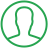# How To Check Data Types In Python

Last Updated : Jul 1, 2023In this article we will show you the solution of how to check data types in python, here we will be using 4 functions, these are : type(), print(isinstance(variable_name, datatype_to_check)), dir() and inspect.getmembers().

Out of these 4 methods the most commonly used method is “type()” function.

## Step By Step Guide On How To Check Data Types In Python :-

Data types are an important concept in any programming language. In Python, data types are used to define the type of data that can be stored and manipulated in a program.

Knowing the data type of a variable or expression can help you determine what operations can be performed on it.

We will learn how to check the data type of a variable or expression in Python using the below codes and steps.

```x = 10
print(type(x))
y = "talkerscode"
print(type(y))
print(isinstance(x, int))
print(dir(x))
import inspect
print(inspect.getmembers(x))
```
1. The first and the most commonly used method to check the data type of a variable or expression in Python is to use the type() function. This is an in built function in python and takes one argument and returns its type. We can see from the code section, if we want to check the type of the variable x, print(type(x)), we will get output as, <class 'int'>, this indicates the type of x, which is 'int' (for datatype as integer).
2. And if we want to check the type of the variable y, print(type(y)), we will get ouput as, <class 'str'>, this indicates the type of y, which is 'str' (for datatype as string).
3. The second way to check the data type of a variable or expression in Python is to use the isinstance() function. This is also an inbuilt function in python, and it takes two arguments: the first argument is the object to be checked, and the second argument is the type to check for. We can see from the code section, if we want to check the variable x is an integer, we can do the print(isinstance(x, int)), this will print out 'True' since x is indeed an integer.
4. The third way to check the data type of a variable or expression in Python is to use the dir() function. The dir() function takes one argument and returns a list of all the attributes and methods associated with the object. We can see from the code section, if we want to check the type of the variable x, we can use print(dir(x)). This will print out a list of all the attributes and methods associated with the integer object. Among these, you will find '__class__', which will indicate the type of x (in this case, 'int').
5. The fourth way to check the data type of a variable or expression in Python is to use the inspect module. The inspect module provides functions for inspecting live objects, such as modules, classes, methods, functions, tracebacks, and code objects. We can see from the code section, if we want to check the type of the variable x, we can use print(inspect.getmembers(x)). This will print out a list of all the attributes and methods associated with the integer object. Among these, you will find '__class__', which will indicate the type of x (in this case, 'int').

## Conclusion :-

In conclusion, we are able to know that there are four ways to check the data type of a variable in Python.

You can use the type() function, the isinstance() function, the dir() function, or the inspect.getmembers(x) with inspect module.

Knowing the data type of a variable or expression can help you determine what operations can be performed on it.

I hope this article on how to check data types in python helps you and the steps and method mentioned above are easy to follow and implement.

##About Anjali

Experienced Computer Programmer with a broad range of experience in technology. Strengths in application development and Object Oriented architecture design, front end programming, usability and multimedia technology. Expert in coding languages such as C, C++ Java, JavaScript, PHP and more.